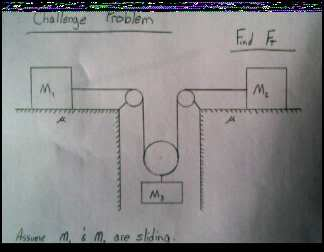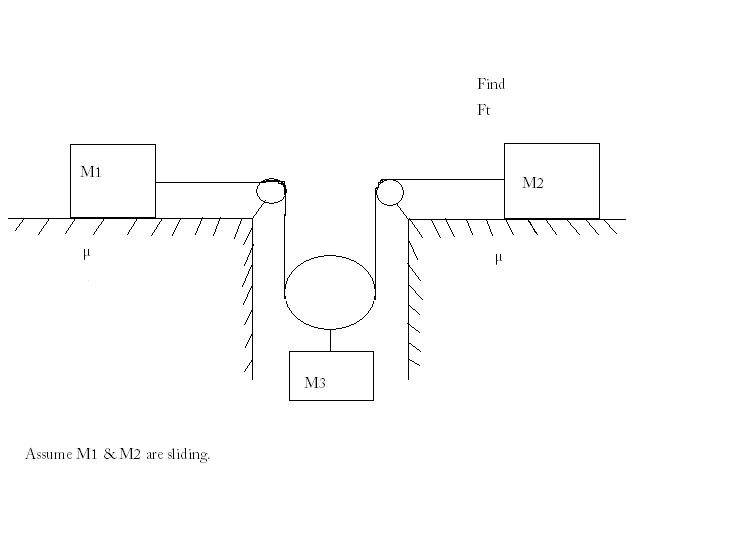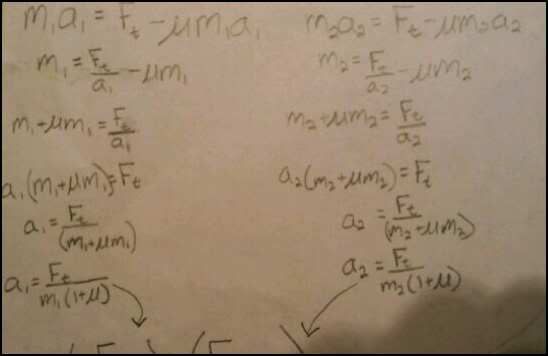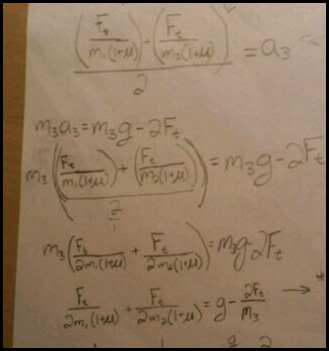# Finding Tensile Force in Pulley Problem

## Homework Statement

See Picture, The problem gives three boxes(M1, M2, and M3) and a few pulleys, no value for the coefficient of friction is given. None of the masses are known, and we are told to assume M1 and M3 are sliding. The objective is to find Ft(if you can't see that well enough i made this)F=ma
Ff=µma

## The Attempt at a Solution

First I started of by drawing free body diagrams for all of the boxes, Using these(and assuming M1 and M2 are sliding towards the middle) I came up with

M1:
FNET=Ft-Ff
M1a=Ft-µM1a
Ft=M1a+µM1a

M2 Would then have the same equations except M1 would be M2 because it also has
FNET=Ft-Ff

(I feel like that might be wrong but I'm not sure)
I'm not sure about the signs on all of those either.

Then I have for M3
FNET=Fg-Ft

At this point I'm not sure what to do, (the lack of values is killing me) I would think putting Ft from the M1 equation into the Ft equation would be a good start, but then everything except mass would cancel wouldn't it? So that doesn't seem right. I feel like I'm going in the right direction and I need to use these equations simultaneously some how but I'm not sure what to do for the next step as I don't see how this is going to get me Ft.

Also I'm starting to think M2 Net force is different
FNET=Ff-Ft (Since the ropes would be moving in opposite directions) and that would also change the equation for M2 to

M2a=µM2a-Ft
Ft=µM2a+M2a

But even if that's right, what would i do with it...?

Any help is appreciated, I'm currently stumped.Doc Al
Mentor
First I started of by drawing free body diagrams for all of the boxes, Using these(and assuming M1 and M2 are sliding towards the middle) I came up with

M1:
FNET=Ft-Ff
M1a=Ft-µM1a
Ft=M1a+µM1a
OK, but be careful to distinguish the accelerations of each mass--they aren't the same. Call this acceleration a1.

M2 Would then have the same equations except M1 would be M2 because it also has
FNET=Ft-Ff

(I feel like that might be wrong but I'm not sure)
I'm not sure about the signs on all of those either.
OK. Use a2.

Then I have for M3
FNET=Fg-Ft
Consider M3 and the pulley as a single object and apply Newton's 2nd law as before. (Careful with the tension force here.)

Key point: How does the acceleration of M3 relate to a1 and a2? Figure that out.

Hint: Set up these equations correctly and you'll have 3 equations and 3 unknowns. (Treat the masses and μ as knowns.)

(You're on the right track.)

Consider M3 and the pulley as a single object and apply Newton's 2nd law as before. (Careful with the tension force here.)

If they are both treated as the same object then it would have two tensile forces on it correct?

Giving you FNET=M3a3-Ft1-Ft2
and a3=g=9.81m/s^2

Both of the tensile forces will be equal I assume so,
M3a=M3g-(2Ft)

Right?

Doc Al
Mentor
If they are both treated as the same object then it would have two tensile forces on it correct?
Right.

Giving you FNET=M3a3-Ft1-Ft2
and a3=g=9.81m/s^2
Well, I wouldn't use a3 to represent g; a3 is the acceleration of M3.

Both of the tensile forces will be equal I assume so,
M3a=M3g-(2Ft)

Right?
Right.

Also I'm not really sure how to go about this part of it, wouldn't it all be equal?
Play around with it, or figure it out by considering that the length of the rope doesn't change. Example: If M1 moves 1 m to the right and M2 moves 1 m to the left, how far does M3 move down?

I Solved for a3 in the net force equation for M3 and got
a3=9.81-(Ft1-Ft2)/M3
then I substituted the values i got for tension for the first and second box earlier and came up with

a3=9.81m/s^2 - ((M1a1+µM1a1)/M3)-((M2a2+µM2a2)/M3)

assuming i did my algebra right...

Is that what you meant by relating all three accelerations?

Im looking at what i said and it makes me think, If a3 = 9.81-2Ft/M3
M1=M2 and a1=a2.
Although what you said earlier to label the accelerations different makes it seem like they shouldn't be equal.

Edit:::

I Think this whole post is going in the wrong direction, read below post

Last edited:
Okay, so... If the Left box moves 1 meter right, and the Right box moves 1 meter left, The middle box moves down 1 meter So, (a1+a2)/2=a3

Doc Al
Mentor
So, (a1+a2)/2=a3
Exactly! This is the constraint equation relating the motion of the three masses. Now you should have all you need to solve for the tension.

(It's worth your time to learn how to derive this a bit more rigorously from the fact that the string length is fixed.)

If you solve for a1 in the first equation and a2 in the second equation

Plug the results into (a1+a2)=a3 and then substitute that into the the equation for M3

Using that solve for Ft

And you end up getting

Ft=[2m1m2m3(1+u)] / [m3(m1+m2) + 4m1m2]

I think i did that right but my teacher said that i will have M1 M2 M3 g and u. when I solve for Ft. My equation is missing the g.
What could i be doing wrong

Doc Al
Mentor
If you solve for a1 in the first equation and a2 in the second equation

Plug the results into (a1+a2)=a3 and then substitute that into the the equation for M3

Using that solve for Ft
OK, except that a3 = (a1 + a2)/2

And you end up getting

Ft=[2m1m2m3(1+u)] / [m3(m1+m2) + 4m1m2]
That cannot be correct since the units don't match. The right hand side has units of mass, not force. (And yes, you should have a g in your answer.)

To find out where you went wrong, show the equations that you used.

Okay, for some reason i accidently substituted g for a1 and a2 on the right of the first two equations, im reworking it now with the correct variables there, and i also see one other mistake...

This time through im using the equations

M1a1=Ft-µM1a1 (1st equation)
M2a2=Ft-µM2a2 (2nd equation)
M3a3=Fg-Ft or M3a3=M3g-Ft (3rd equation)
a3 = (a1 + a2)/2 (4th equation) that's what I meant last time I just forgot to write the divided by 2 as I was typing it fast sorry

Ill edit this post when i get an answer with these equations

Doc Al
Mentor
M3a3=Fg-Ft or M3a3=M3g-Ft (3rd equation)
Careful: The tension pulls up on M3 twice.

Careful: The tension pulls up on M3 twice.

Right. thats what i have written down, forgot that two when i typed it in. Other than that do all the equations look good? I'm still working on the algebra to solve it for Ft.

Doc Al
Mentor
Other than that do all the equations look good?
Yep; looks good.

I feel like im really bad at algebra right now, so far i have

1/2m1(1+u)+1/2m2(1+u)=g/Ft-2/m3

Everything i do to this makes it feel like im going backwards, if i multiply by ones on the right side to get a common denominator it takes that straight back to m3g-2Ft

Any suggestions on the next step? I'm still tryingDoc Al
Mentor
Not sure how you ended up with Ft in the denominator.

Why don't you follow the same approach you described in post #8?

i had Ft/2m1(1+u) + Ft/2m2(1+u)=g-2Ft/m3 and i tried dividing by Ft

thats how it got on the bottom

With that i continued to 1/M1+1/M2=2g(1+u)/Ft - 4(1+u)/M3

I can multiply by Ft here to get it back on top on both the fractions on the left and the last fraction

And originally i solved for a1, a2, and substituting that into (a1+a2)/2=a3 and then substituted a3 into m3a3=m3g-2Ft

Thats where i started trying to get Ft

Doc Al
Mentor
i had Ft/2m1(1+u) + Ft/2m2(1+u)=g-2Ft/m3 and i tried dividing by Ft
Since you want to solve for Ft, don't divide by it. Instead, start by collecting all terms with Ft on the left side of the equation. Then just factor out the Ft and simplify the result.

I think i got it :surprised

Ft=2gm1m2m3(1+u)/(m2m3-m1m3+4m1m2(1+u))

Is that correct? god i hope so

Edit:: I think i can pull out an M3 from the denominator and make it m3(m2-m1)+4m1m2(1+u)

Last edited:
Doc Al
Mentor
You are very close but I think you made an error somewhere. (As a sanity check, you might want to consider the special case where all masses are equal and there's no friction. In that case the tension will be mg/3.)

Im trying to set all the masses equal and set my u to 0, and i cant find out where i messed up.

I substituted like that at the point where i got my last equation and it came to 2gm/5.

...Can you see anything i'm doing wrong?

from this point i would get the Ft on the left and factor/solve

Edit:: Wow I feel stupid it does work out to gm/3... For some reason i thought 4+1+1 was equal to 5 >.<. I'm working it out again

Last edited:
I think i got it :surprised

Ft=2gm1m2m3(1+u)/(m2m3-m1m3+4m1m2(1+u))

Is that correct? god i hope so

Edit:: I think i can pull out an M3 from the denominator and make it m3(m2-m1)+4m1m2(1+u)

Okay, i went back through it and, somehow i turned a positive into a negative in the middle of all my algebra for no reason. So now i have the same thing except

Ft=2gm1m2m3(1+u)/(m2m3+m1m3+4m1m2(1+u))

Doc Al
Mentor
Okay, i went back through it and, somehow i turned a positive into a negative in the middle of all my algebra for no reason. So now i have the same thing except

Ft=2gm1m2m3(1+u)/(m2m3+m1m3+4m1m2(1+u))
That's almost what I get. How did you get the (1+μ) in the denominator?

Unfortunately, I missed a few errors (typos, I presume) in your earlier equations:
M1a1=Ft-µM1a1 (1st equation)
M2a2=Ft-µM2a2 (2nd equation)
The friction force terms should be μMg, not μMa.

(Algebra's a pain in the butt, ain't it?)

Unfortunately, I missed a few errors (typos, I presume) in your earlier equations:

The friction force terms should be μMg, not μMa.

(Algebra's a pain in the butt, ain't it?)

Darn, I thought I was gonna be the first to catch that oneUnfortunately, I missed a few errors (typos, I presume) in your earlier equations:

The friction force terms should be μMg, not μMa.

Unfortunately I used, μMa meaning I probably got it wrong. I turned it in today so i don't have any of the work with me. When I get it back (Wednesday maybe?) I'll go back over and finish out the problem the right way.

Hopefully I'll get partial extra credit for what I had.